## RS Aggarwal Class 9 Solutions Chapter 14 Statistics Ex 14B

These Solutions are part of RS Aggarwal Solutions Class 9. Here we have given RS Aggarwal Solutions Class 9 Chapter 14 Statistics Ex 14B.

Other Exercises

Question 1.
Solution:
We shall take the game along x-axis and number of students along y-axis.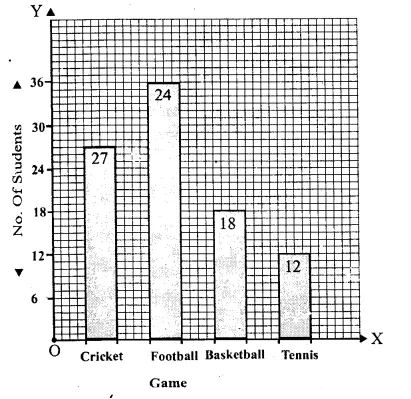Question 2.
Solution:
We shall take the time on x-axis and temperature (in °C) on y-axis.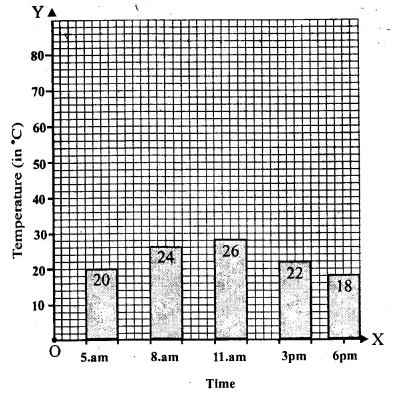Question 3.
Solution:
We shall take name of vehicle on x-axis and velocity (in km/hr) on y-axisQuestion 4.
Solution:
We shall take sports on x-axis and number of students on y-axis.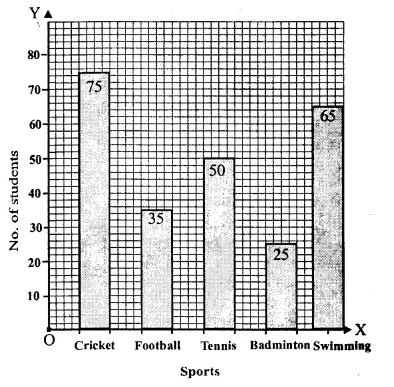Question 5.
Solution:
We shall take years on x-axis and number of students on y-axis.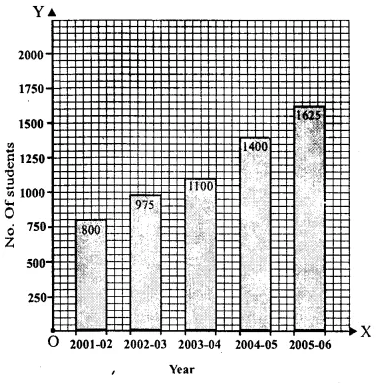Question 6.
Solution:
We shall take years on x-axis and number of students on y-axis.Question 7.
Solution:
We shall take years on x-axis and number of students on y-axis.Question 8.
Solution:
We shall take years on x-axis and number of students on y-axis.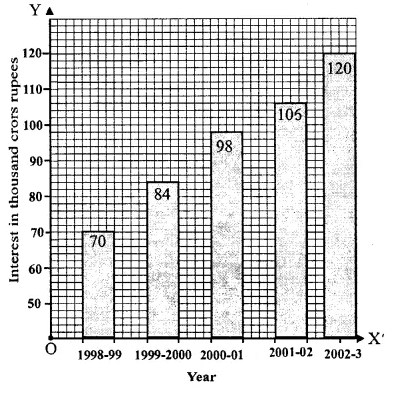Question 9.
Solution:
We shall take years on x-axis and number of students on y-axis.Question 10.
Solution:
We shall take years on x-axis and number of students on y-axis.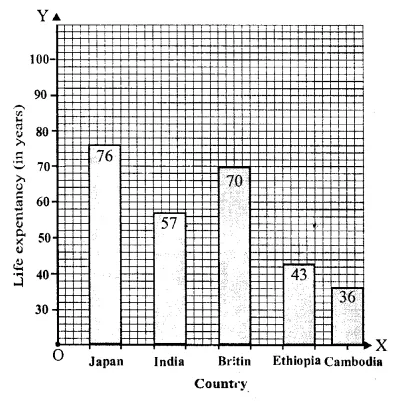Question 11.
Solution:
We shall take week on x-axis and Rate per 10g (in Rs.) on y-axis.Question 12.
Solution:
We shall take mode of transport on x-axis and number of students on y-axis.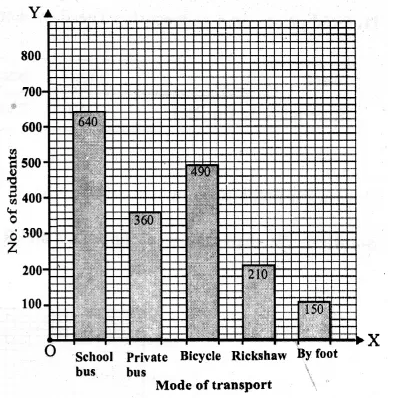Question 13.
Solution:
We see from the graph that
(i) It shows the marks obtained by a student in various subjects.
(ii) The student is very well in mathematics.
(iii) The student is very’ poor, in Hindi.
(iv) Average marks
= $$\frac { 60+35+75+50+60 }{ 5 }$$ (Here x = 5)
= $$\frac { 280 }{ 5 }$$
= 56 marksHope given RS Aggarwal Solutions Class 9 Chapter 14 Statistics Ex 14B are helpful to complete your math homework.

If you have any doubts, please comment below. Learn Insta try to provide online math tutoring for you.